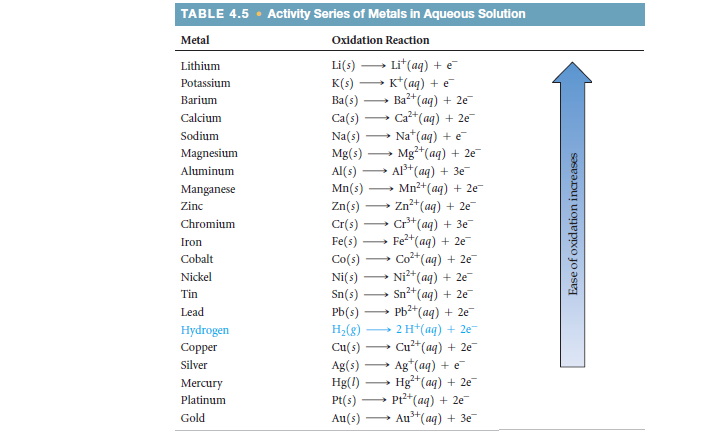Problem: Using the activity series (Table 4.5), write balanced chemical equations for the following reactions. If no reaction occurs, simply write NR. (a) Iron metal is added to a solution of copper(II) nitrate

FREE Expert Solution
83% (297 ratings)
Problem Details

Using the activity series (Table 4.5), write balanced chemical equations for the following reactions. If no reaction occurs, simply write NR. (a) Iron metal is added to a solution of copper(II) nitrate